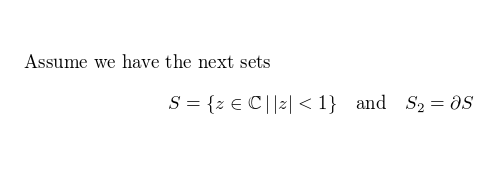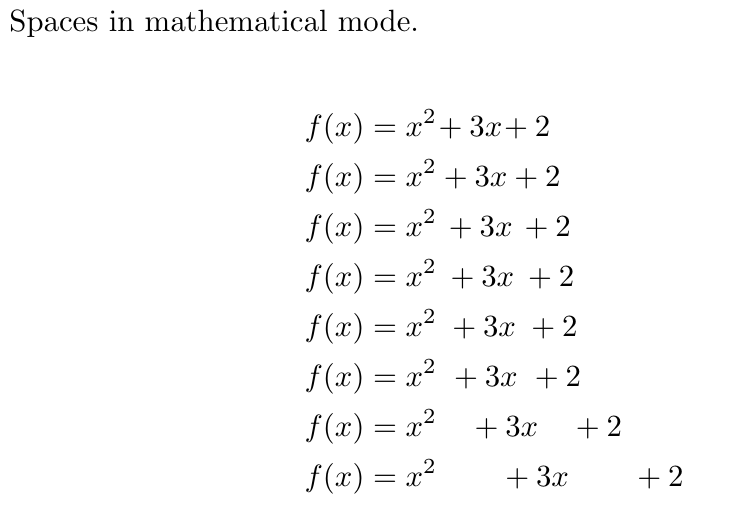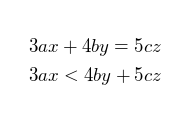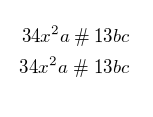Showing first {{hits.length}} results of {{hits_total}} for {{searchQueryText}}{{hits.length}} results for {{searchQueryText}}

Ничего не найдено

In mathematical mode characters are spaced as if they were part of a single word, regardless of the actual space you insert. This article explains how to insert spaces of different lengths in mathematical mode. Contents 1 Introduction 2 Spaces 3 Operators spacing 4 User-defined binary and relational operators 5 Reference guide 6 Further reading Introduction Spacing in maths mode is useful in several situations, let's see an example: Assume we have the next sets $S = \{ z \in \mathbb{C}\, |\, |z| < 1 \} \quad \textrm{and} \quad S_2=\partial{S}$As you see in this example, a mathematical text can be explicitly spaced by means of some special commands   Open an example in Overleaf Spaces The spacing depends on the command you insert, the example below contains a complete list of spaces and how they look like. Spaces in mathematical mode. \begin{align*} f(x) &= x^2\! +3x\! +2 \\ f(x) &= x^2+3x+2 \\ f(x) &= x^2\, +3x\, +2 \\ f(x) &= x^2\: +3x\: +2 \\ f(x) &= x^2\; +3x\; +2 \\ f(x) &= x^2\ +3x\ +2 \\ f(x) &= x^2\quad +3x\quad +2 \\ f(x) &= x^2\qquad +3x\qquad +2 \end{align*}Check the reference guide for a description of the commands. Note: to see a description of the align* environment see Aligning equations with amsmath   Open an example in Overleaf Operators spacing Spacing around operators and relations in math mode are governed by specific skip lengths: \thinmuskip (by default it is equal to 3 mu) \medmuskip (by default it is equal to 4 mu) \thickmuskip (by default it is equal to 5 mu) \begin{align*} 3ax+4by=5cz\\ 3ax<4by+5cz \end{align*}For relationnal operators, such as < , > and =, LaTeX establishes \thickmuskip space. But for binary operators such as +, - and x, the \medmuskip space is set. The difference is almost unnoticeable.   Open an example in Overleaf User-defined binary and relational operators You can force the spacing used in binary or relational operators, so you can define your own. \begin{align*} 34x^2a \mathbin{\#} 13bc \\ 34x^2a \mathrel{\#} 13bc \end{align*}The previous example sets a particular spacing before and after # by using \mathrel (relational) and \mathbin (binary) commands.   Open an example in Overleaf Reference guide Description of spacing commands LaTeX code Description \quad space equal to the current font size (= 18 mu) \, 3/18 of \quad (= 3 mu) \: 4/18 of \quad (= 4 mu) \; 5/18 of \quad (= 5 mu) \! -3/18 of \quad (= -3 mu) \ (space after backslash!) equivalent of space in normal text \qquad twice of \quad (= 36 mu) Further reading For more information see Mathematical expressions Brackets and Parentheses Aligning equations with amsmath Display style in math mode List of Greek letters and math symbols Mathematical fonts The not so short introduction to LaTeX2ε